### 2 安装与调试Tensorboard

#### 2.1 安装与启动

xxxxxxxxxx11pip install tensorflow

xxxxxxxxxx11tensorboard --logdir=runs

xxxxxxxxxx11TensorBoard 1.15.0 at http://localhost:6006/ (Press CTRL+C to quit)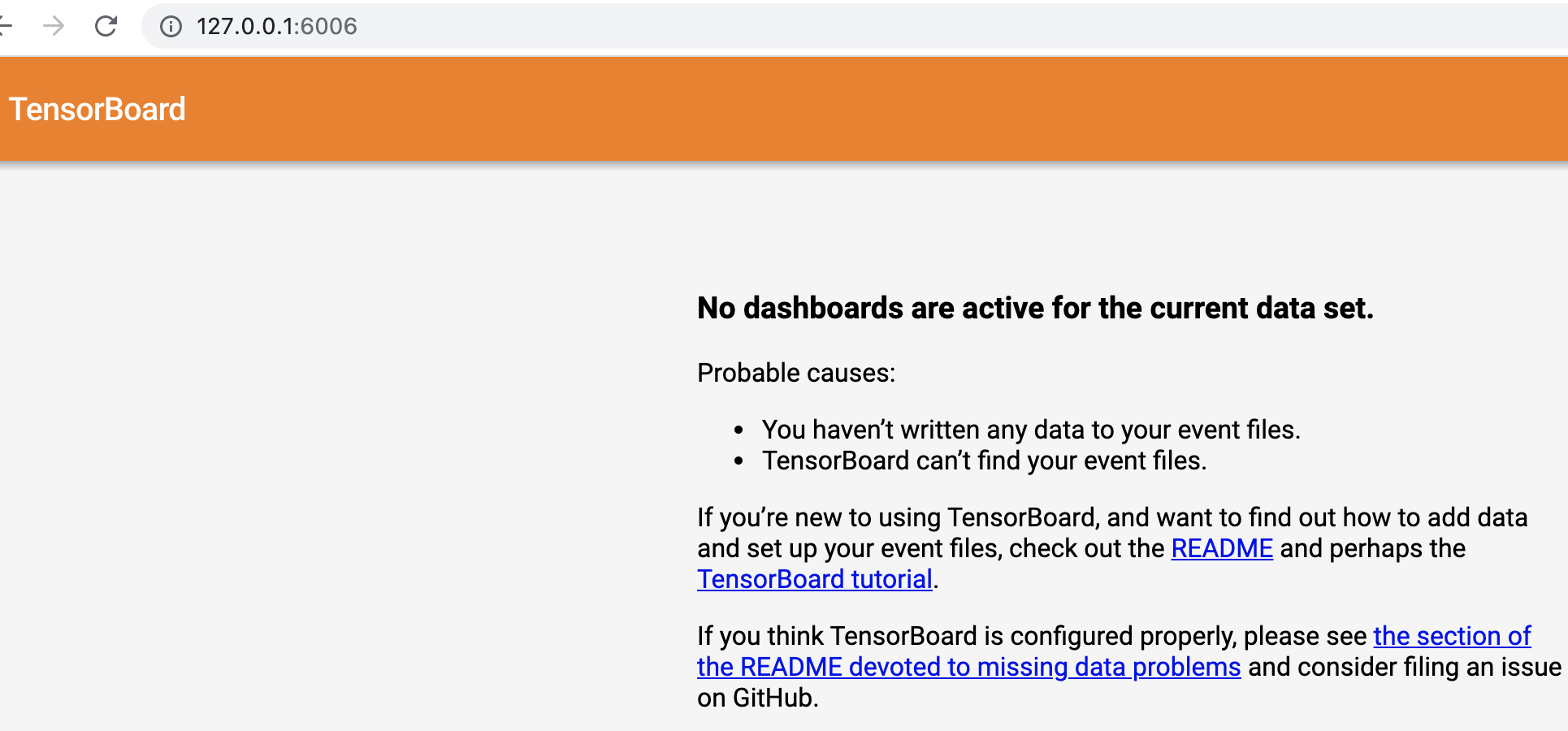xxxxxxxxxx11tensorboard --logdir=runs --host 0.0.0.0

#### 2.2 远程连接

##### 2.2.1 通过IP直接访问

xxxxxxxxxx11http://IP:6006

• 公网主机

• 在后台的安全策略里面查看一下6006这个端口有没有被打开，如果没有则需要打开；
• 查看IP是否为公网IP，在主机的后台管理页面可以看到。
• 局域网主机

• 查看本地电脑是否和主机处于同一网段；

• 查看主机的6006端口是否打开，如果没有可以通过如下命令打开

xxxxxxxxxx41firewall-cmd --zone=public --list-ports # 查看已开放端口2firewall-cmd --zone=public --add-port=5672/tcp --permanent #开放5672端口3firewall-cmd --zone=public --remove-port=5672/tcp --permanent #关闭56724firewall-cmd --reload   # 配置立即生效
##### 2.2.2 通过SSH转发访问

• 命令行终端

如果你的命令行终端支持SSH命令（例如较新的Windows10 的CMD或者Linux等）的话，那么可以直接通过下面这一条命令来进行连接：

xxxxxxxxxx11ssh -L 16006:127.0.0.1:6006 username@ip

这条命令的含义就是将服务器上6006端口的信息通过SSH转发到本地的16006端口，其中16006是本地的任意端口（无限制），只要不和本地应用有冲突就行；后面则是对应的用户名和IP。

上述命令连接成功并根据2.1节中的命令启动Tensorboard之后，在本地通过浏览器打开如下地址即可：

xxxxxxxxxx11http://127.0.0.1:16006
• Xshell工具

如果你的电脑终端不支持SSH命令的话，那么你还可以通过Xshell工具来实现SSH反向代理。首先你需要安装好Xshell工具，没有的话可以参考这篇文章进行安装。在安装完成后按照如下步骤配置即可：

第一步：新建连接

如图2所示，点击新建连接。图 2. 新建连接（一）

然后再根据图3所示的界面配置主机信息。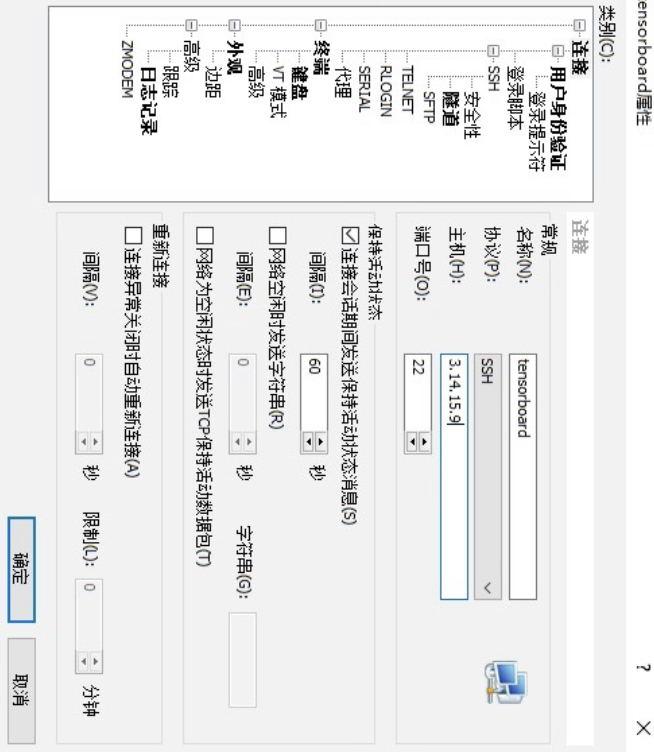图 3. 新建连接（二）

第二步：配置代理

进一步，如图4所示点击侧边栏的隧道，并点击右侧的添加按钮。图 4. 配置代理（一）

接着再根据图5所示的示例进行端口代理配置。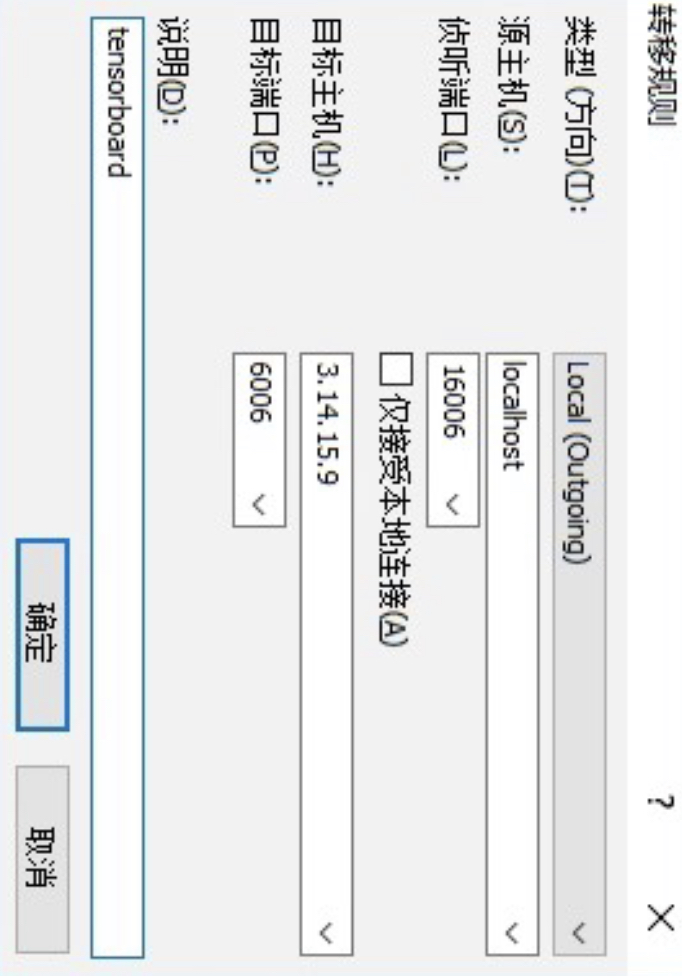图 5. 配置代理（二）

配置完成后点击图6中的确认即可。图 6. 配置代理（三）

完成上述两步配置之后，再双击刚刚这个新建的连接输入用户名和密码之后即可登录到主机并对相应的端口进行了监听与转发。最后我们同样只需要先在当前的命令行中启动Tensorboard，再在本地浏览器中通过如下地址进行访问即可：

xxxxxxxxxx11http://127.0.0.1:16006

### 3 使用Tensoboard

#### 3.1 add_scalar方法

xxxxxxxxxx91from torch.utils.tensorboard import SummaryWriter2if __name__ == '__main__':     3    writer = SummaryWriter(log_dir="runs/result_1", flush_secs=120)4    for n_iter in range(100):5        writer.add_scalar(tag='Loss/train',6                          scalar_value=np.random.random(),7                          global_step=n_iter)8        writer.add_scalar('Loss/test', np.random.random(), n_iter)9    writer.close()

xxxxxxxxxx31tensorboard --logdir=runs2
3TensorBoard 1.15.0 at http://localhost:6006/ (Press CTRL+C to quit)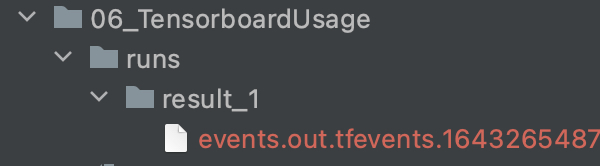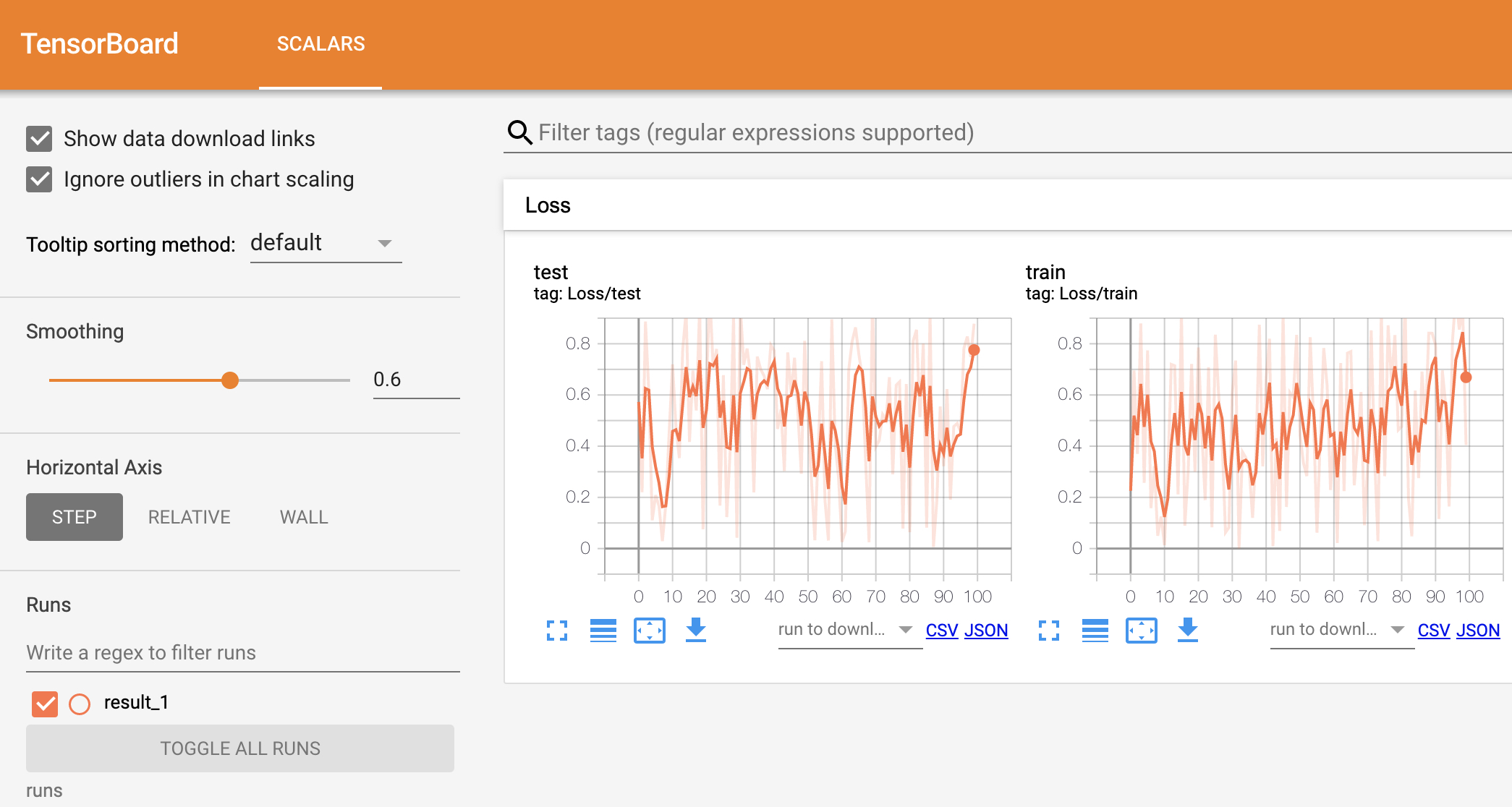#### 3.2 add_graph方法

xxxxxxxxxx51import torchvision2def add_graph(writer):3    img = torch.rand([1, 3, 64, 64], dtype=torch.float32)4    model = torchvision.models.AlexNet(num_classes=10)5    writer.add_graph(model, input_to_model=img)  # 类似于TensorFlow 1.x 中的fed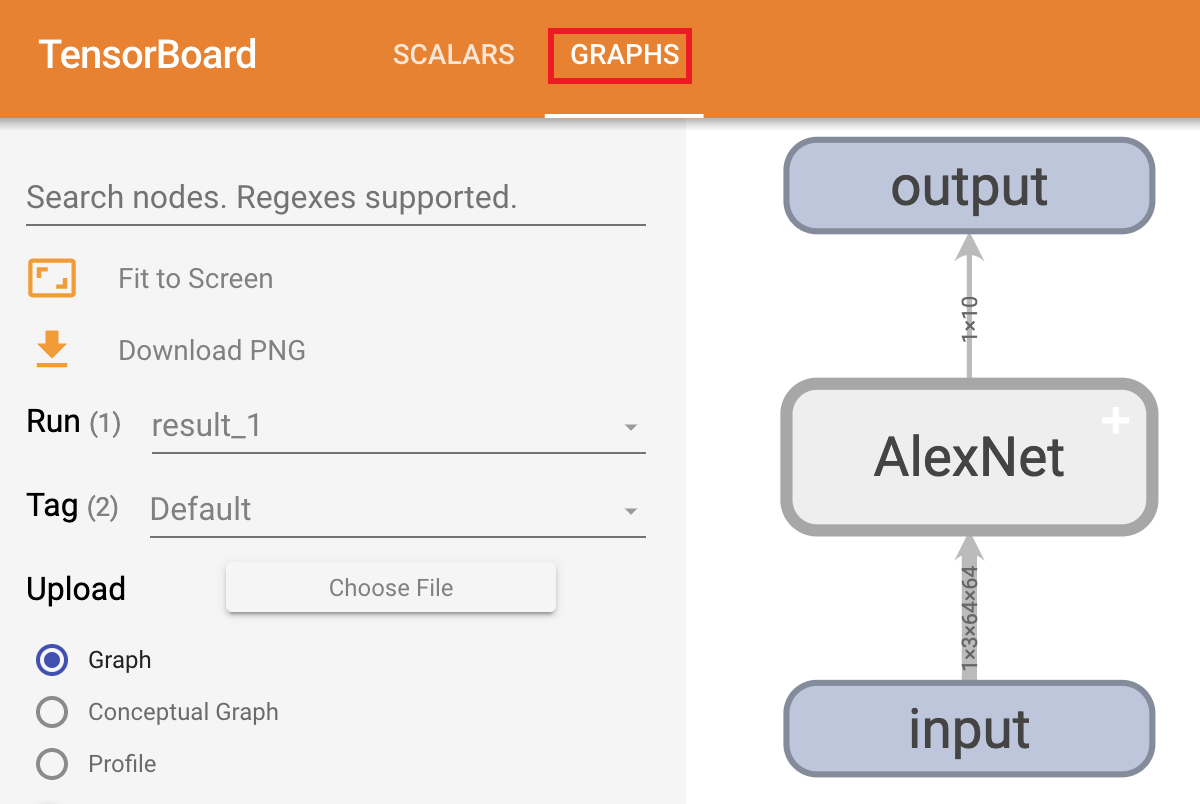#### 3.3 add_scalars方法

xxxxxxxxxx211def add_scalars(writer):2    r = 53    for i in range(100):4        writer.add_scalars(main_tag='scalars1/P1',5                           tag_scalar_dict={'xsinx': i * np.sin(i / r),6                                            'xcosx': i * np.cos(i / r),7                                            'tanx': np.tan(i / r)},8                           global_step=i)9        writer.add_scalars('scalars1/P2',10                           {'xsinx': i * np.sin(i / (2 * r)),11                            'xcosx': i * np.cos(i / (2 * r)),12                            'tanx': np.tan(i / (2 * r))}, i)13        writer.add_scalars(main_tag='scalars2/Q1',14                           tag_scalar_dict={'xsinx': i * np.sin((2 * i) / r),15                                            'xcosx': i * np.cos((2 * i) / r),16                                            'tanx': np.tan((2 * i) / r)},17                           global_step=i)18        writer.add_scalars('scalars2/Q2',19                           {'xsinx': i * np.sin(i / (0.5 * r)),20                            'xcosx': i * np.cos(i / (0.5 * r)),21                            'tanx': np.tan(i / (0.5 * r))}, i)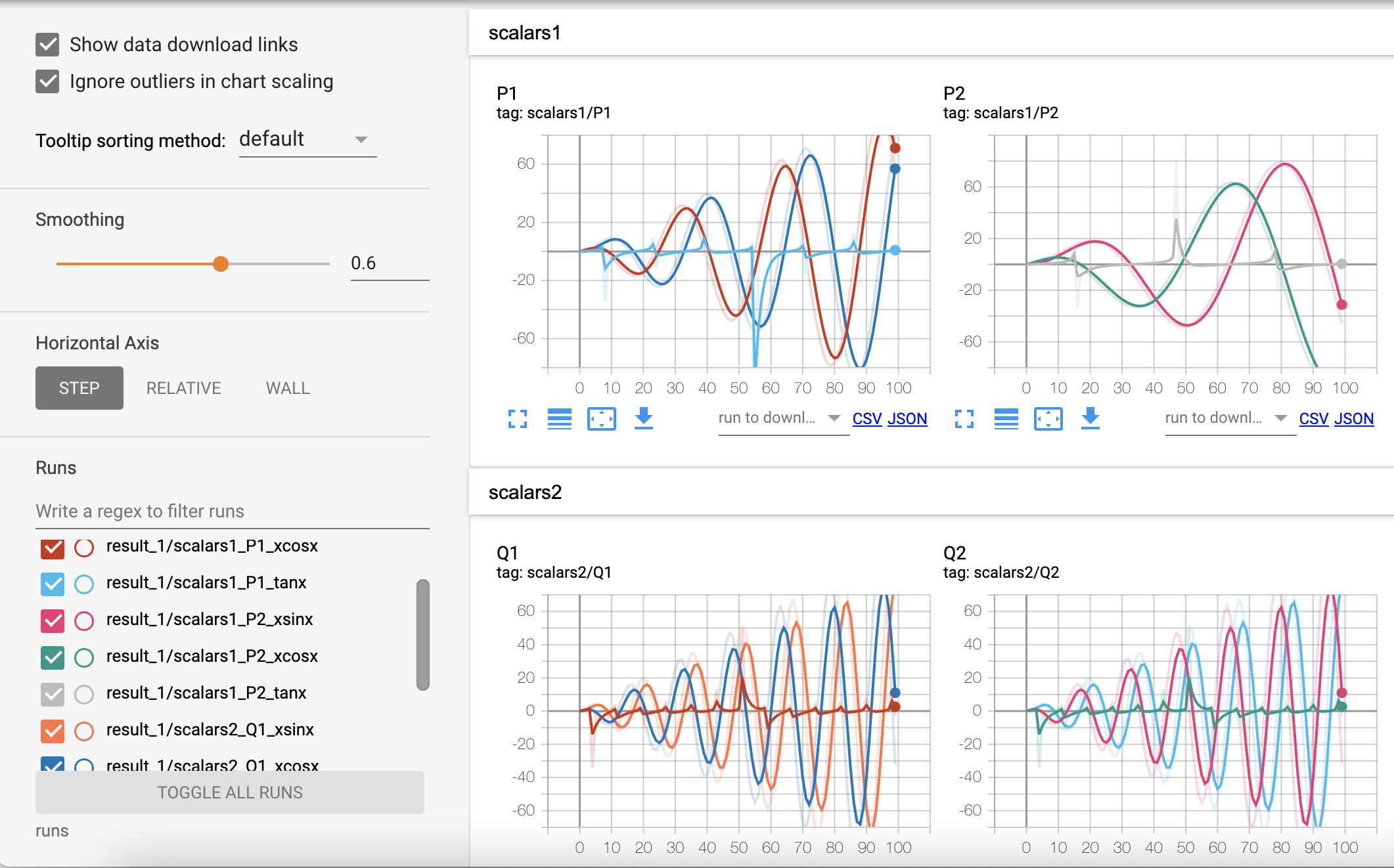#### 3.4 add_histogram方法

xxxxxxxxxx51def add_histogram(writer):2    for i in range(10):3        x = np.random.random(1000)4        writer.add_histogram('distribution centers/p1', x + i, i)5        writer.add_histogram('distribution centers/p2', x + i * 2, i)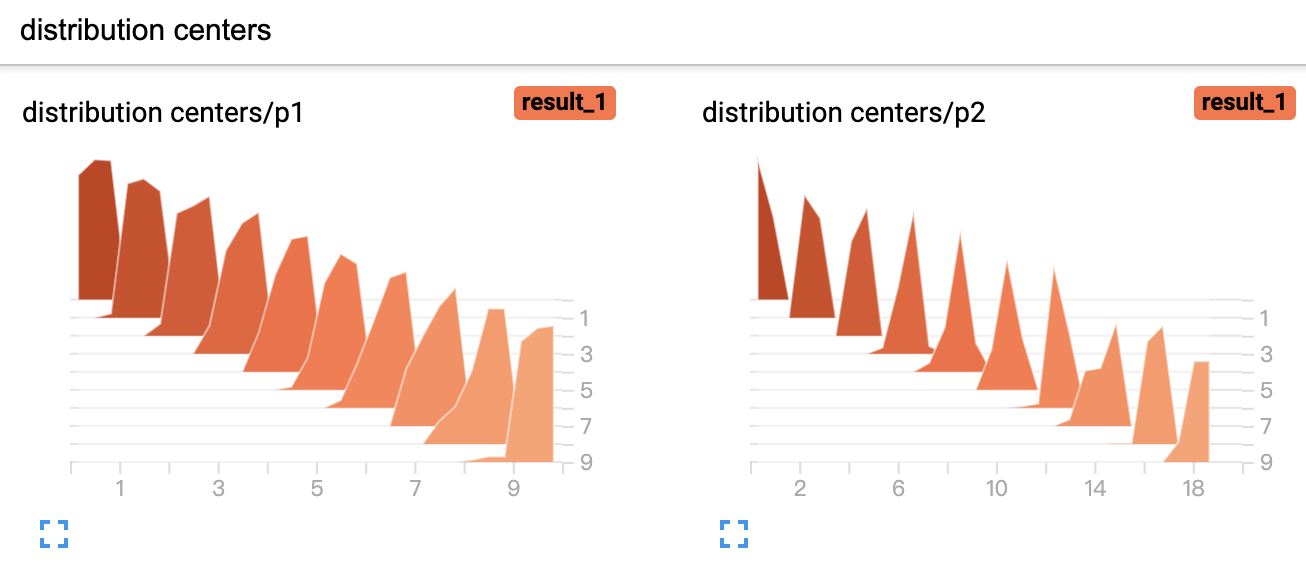#### 3.5 add_image方法

add_image方法是用来可视化相应的像素矩阵，例如本地图片，或者是特征图等

xxxxxxxxxx91def add_image(writer):2    from PIL import Image3    img1 = np.random.randn(1, 100, 100)4    writer.add_image('img/imag1', img1)5    img2 = np.random.randn(100, 100, 3)6    writer.add_image('img/imag2', img2, dataformats='HWC')7    img = Image.open('./dufu.png')8    img_array = np.array(img)9    writer.add_image('local/dufu', img_array, dataformats='HWC')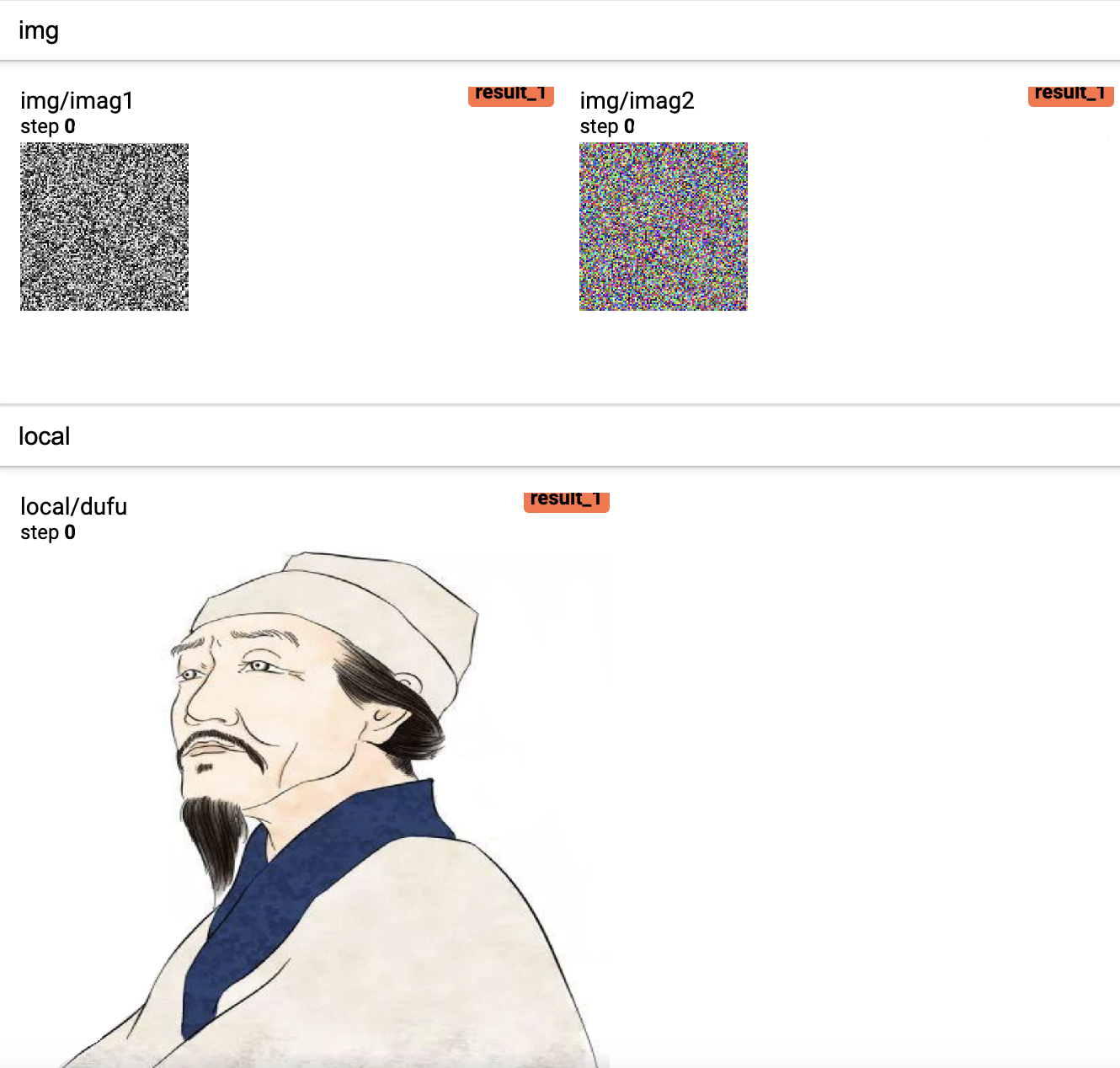#### 3.6 add_images方法

xxxxxxxxxx81def add_images(writer):2    img1 = np.random.randn(8, 100, 100, 1)3    writer.add_images('imgs/imags1', img1, dataformats='NHWC')4    img2 = np.zeros((16, 3, 100, 100))5    for i in range(16):6        img2[i, 0] = np.arange(0, 10000).reshape(100, 100) / 10000 / 16 * i7        img2[i, 1] = (1 - np.arange(0, 10000).reshape(100, 100) / 10000) / 16 * i8    writer.add_images('imgs/imags2', img2)  # Default is :math:(N, 3, H, W)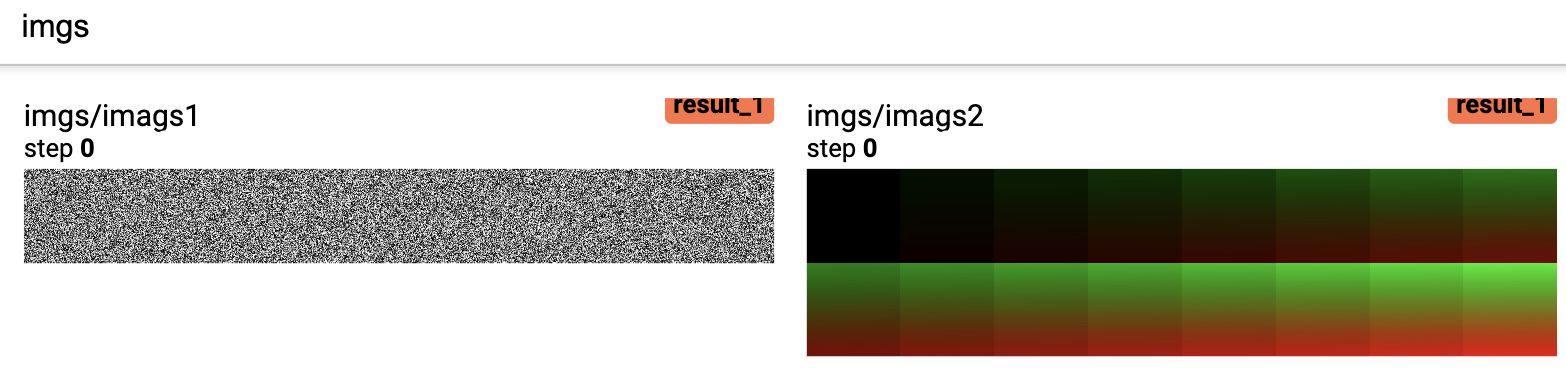#### 3.7 add_figure方法

xxxxxxxxxx91def add_figure(writer):2    fig = plt.figure(figsize=(5, 4))3    ax = fig.add_axes([0.12, 0.1, 0.85, 0.8])4    xx = np.arange(-5, 5, 0.01)5    ax.plot(xx, np.sin(xx), label="sin(x)")6    ax.legend()7    fig.suptitle('Sin(x) figure\n\n', fontweight="bold")8    writer.add_figure("figure", fig, 4)9    # plt.show()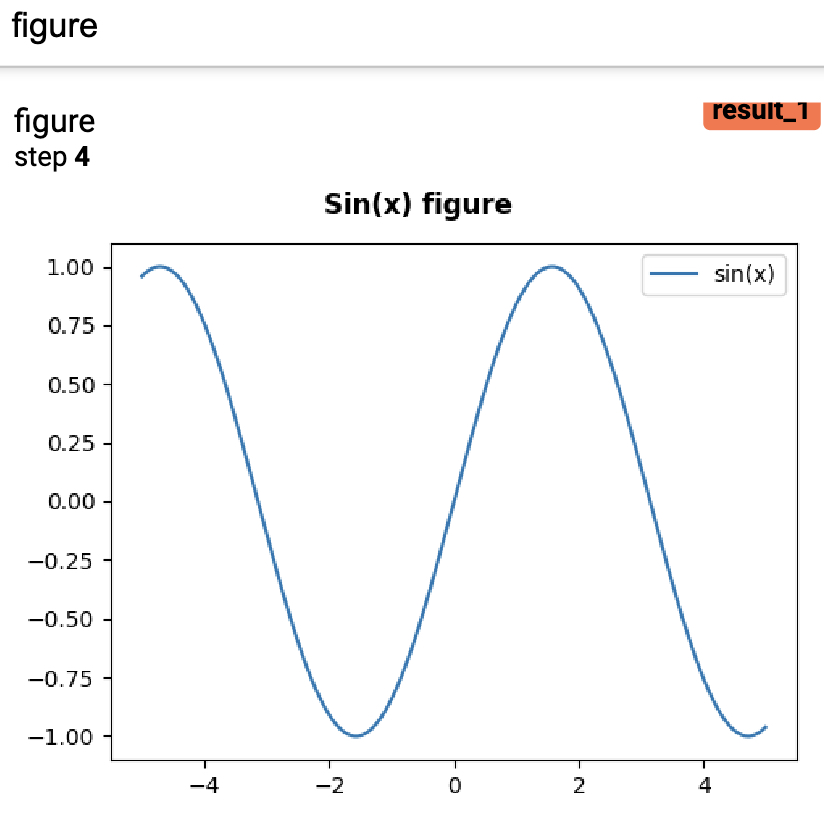xxxxxxxxxx111def add_figures(writer, images, labels):2    text_labels = ['t-shirt', 'trouser', 'pullover', 'dress', 'coat',3                   'sandal', 'shirt', 'sneaker', 'bag', 'ankle boot']4    labels = [text_labels[int(i)] for i in labels]5    fit, ax = plt.subplots(len(images) // 5, 5, figsize=(10, 2 * len(images) // 5))6    for i, axi in enumerate(ax.flat):7        image, label = images[i].reshape([28, 28]).numpy(), labels[i]8        axi.imshow(image)9        axi.set_title(label)10        axi.set(xticks=[], yticks=[])11    writer.add_figure("figure", fit)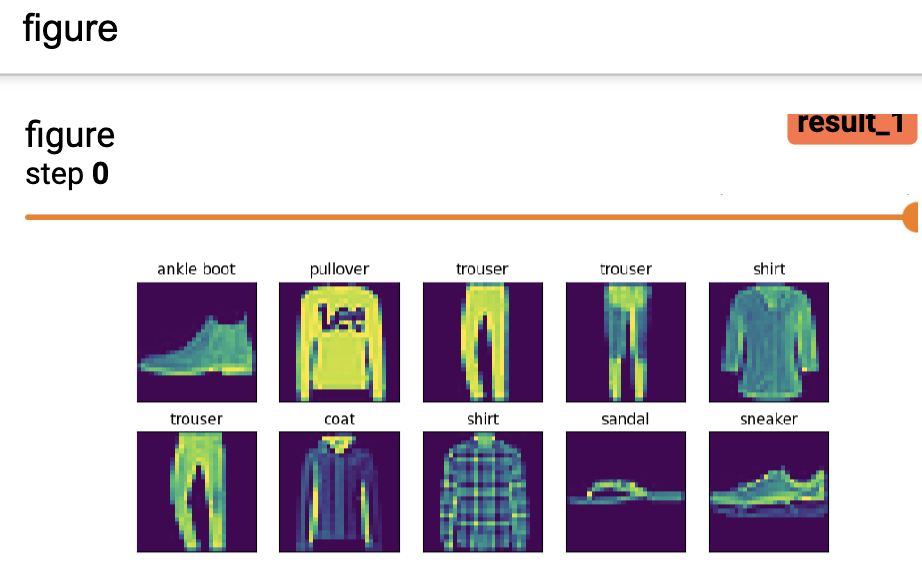#### 3.8 add_pr_curve方法

add_pr_curve这个方法是用来在训练过程中可视化Precision-Recall曲线，即观察在不同阈值下精确率与召回率的平衡情况。更多关于Precision-Recall曲线内容的介绍可以参考文章详解机器学习中的Precision-Recall曲线。用法示例如下所示：

xxxxxxxxxx231def add_pr_curve(writer):2    from sklearn.linear_model import LogisticRegression3    from sklearn.preprocessing import label_binarize4    def get_dataset():5        from sklearn.datasets import load_iris6        from sklearn.model_selection import train_test_split7        x, y = load_iris(return_X_y=True)8        random_state = np.random.RandomState(2020)9        n_samples, n_features = x.shape10        # 为数据增加噪音维度以便更好观察pr曲线11        x = np.concatenate([x, random_state.randn(n_samples, 100 * n_features)], axis=1)12        x_train, x_test, y_train, y_test = train_test_split(x, y, test_size=0.5,13                                                            random_state=random_state)14        return x_train, x_test, y_train, y_test15
16    x_train, x_test, y_train, y_test = get_dataset()17    model = LogisticRegression(multi_class="ovr")18    model.fit(x_train, y_train)19    y_scores = model.predict_proba(x_test)  # shape: (n,3)20
21    b_y = label_binarize(y_test, classes=[0, 1, 2])  # shape: (n,3)22    for i in range(3):23        writer.add_pr_curve(f"pr_curve/label_{i}", b_y[:, i], y_scores[:, i], global_step=1)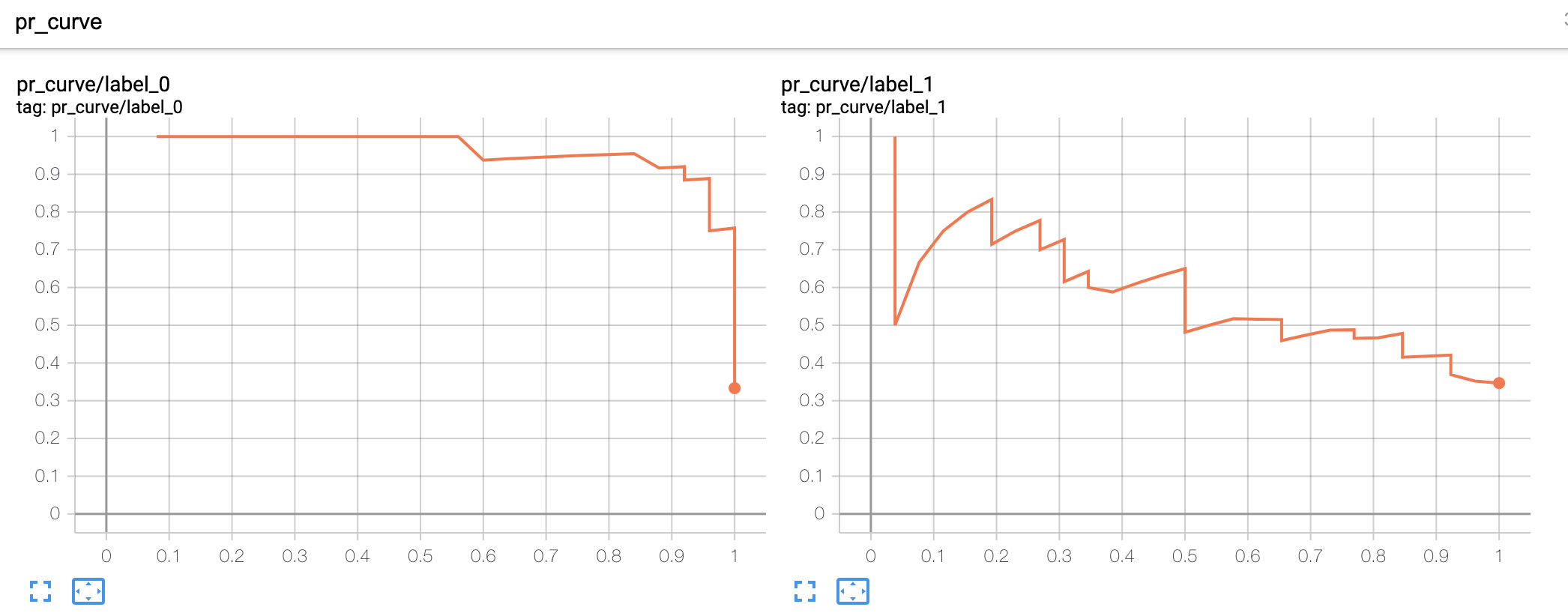#### 3.9 add_embedding方法

mat：用来指定可视化结果中每个点的坐标，形状为$\left(N,D\right)$$(N, D)$不能为空，例如对词向量可视化时mat就是词向量矩阵，图片分类时mat可以是分类层的输出结果；

metadata：用来指定每个点对应的标签信息，是一个包含$N$$N$个元素的字符串列表，为空时则默认为['1','2',....,'N'];

label_img：用来指定每个点对应可视化信息，形状为$\left(N,C,H,W\right)$$(N, C, H, W)$，可以为空，例如图片分类时label_img就是每一张真实图片的可视化结果。

xxxxxxxxxx191def add_embedding(writer):2    import tensorflow as tf3    import tensorboard as tb4    tf.io.gfile = tb.compat.tensorflow_stub.io.gfile5    import keyword6    import torch7    # 随机生成100个标签信息8    meta = []9    while len(meta) < 100:10        meta = meta + keyword.kwlist  # get some strings11    meta = meta[:100]12    for i, v in enumerate(meta):13        meta[i] = v + str(i)14    # 随机生成100个标签图片15    label_img = torch.rand(100, 3, 10, 32)16    for i in range(100):17        label_img[i] *= i / 100.018    data_points = torch.randn(100, 5)  # 随机生成100个点19    writer.add_embedding(mat=data_points, metadata=meta, label_img=label_img, global_step=1)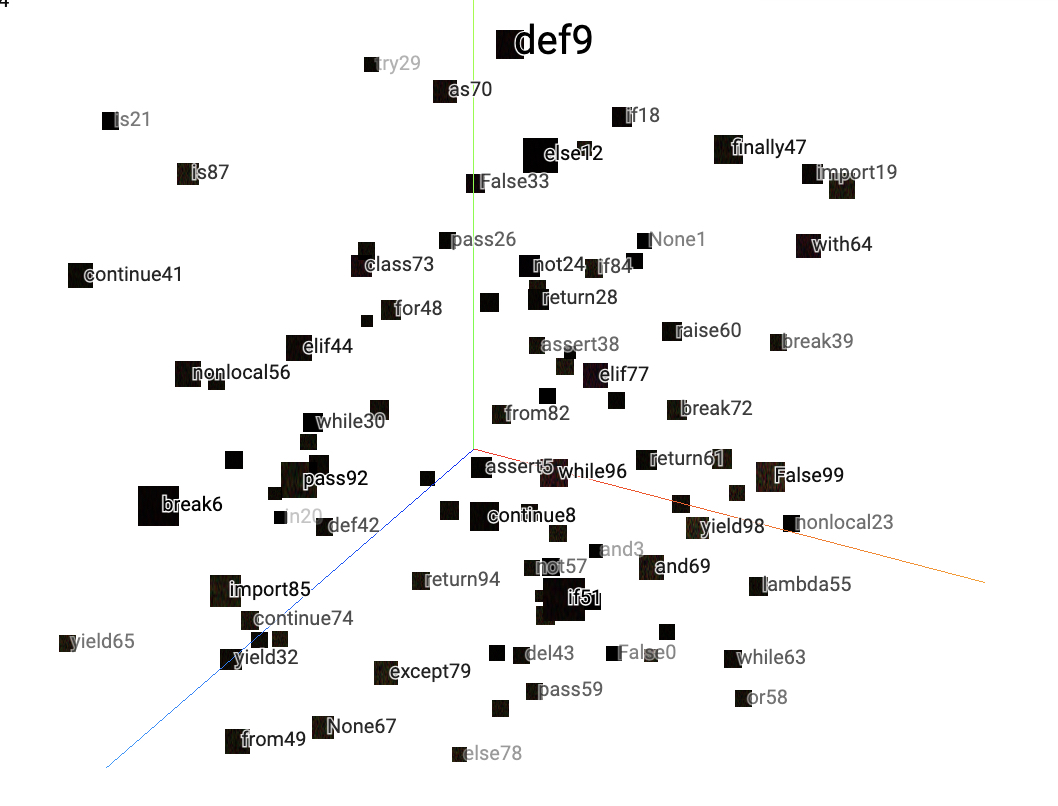### 4 使用实例

#### 4.1 定义模型

xxxxxxxxxx301import torch.nn as nn2
3class LeNet5(nn.Module):4    def __init__(self, ):5        super(LeNet5, self).__init__()6        self.conv = nn.Sequential(  # [n,1,28,28]7            nn.Conv2d(1, 6, 5, padding=2),  # in_channels, out_channels, kernel_size8            nn.ReLU(),  # [n,6,24,24]9            nn.MaxPool2d(2, 2),  # kernel_size, stride  [n,6,14,14]10            nn.Conv2d(6, 16, 5),  # [n,16,10,10]11            nn.ReLU(),12            nn.MaxPool2d(2, 2))  # [n,16,5,5]13
14        self.fc = nn.Sequential(15            nn.Flatten(),16            nn.Linear(16 * 5 * 5, 120),17            nn.ReLU(),18            nn.Linear(120, 84),19            nn.ReLU(),20            nn.Linear(84, 10))21
22    def forward(self, img, labels=None):23        output = self.conv(img)24        logits = self.fc(output)25        if labels is not None:26            loss_fct = nn.CrossEntropyLoss(reduction='mean')27            loss = loss_fct(logits, labels)28            return loss, logits29        else:30            return logits

#### 4.2 定义分类模型

• 数据集构造

首先，我们需要构造训练模型时使用到的数据集，这里掌柜还是以FashionMNIST为例进行示例。构造代码如下所示：

xxxxxxxxxx151text_labels = ['t-shirt', 'trouser', 'pullover', 'dress', 'coat',2               'sandal', 'shirt', 'sneaker', 'bag', 'ankle boot']3
4def load_dataset(batch_size=64):5    mnist_train = torchvision.datasets.FashionMNIST(root='~/Datasets/FashionMNIST',6                                                    train=True, download=True,7                                                    transform=transforms.ToTensor())8    mnist_test = torchvision.datasets.FashionMNIST(root='~/Datasets/FashionMNIST',9                                                   train=False, download=True,10                                                   transform=transforms.ToTensor())11    train_iter = torch.utils.data.DataLoader(mnist_train,batch_size=batch_size,12                                             shuffle=True,num_workers=1)13    test_iter = torch.utils.data.DataLoader(mnist_test,batch_size=batch_size,14                                            shuffle=True,num_workers=1)15    return train_iter, test_iter

在上述代码中，第1-2行为定义的每个标签序号所对应的标签名，用于在使用add_embedding可视化预测结果时展示每个样本的标签名称；第5-15行则是分别构造训练集和测试集的DataLoader对象。

• 定义分类模型

进一步，我们需要定义一个类来实现模型的整个训练和推理过程。首先定义这个类的初始化方法，代码如下：

xxxxxxxxxx111class MyModel:2    def __init__(self,3                 batch_size=64,4                 epochs=3,5                 learning_rate=0.01):6        self.batch_size = batch_size7        self.epochs = epochs8        self.learning_rate = learning_rate9        self.model_save_path = 'model.pt'10        self.device = torch.device('cuda:0' if torch.cuda.is_available() else 'cpu')11        self.model = LeNet5()
• 定义评估方法

在这里，我们先定义一个评估函数，用来计算在测试集上模型的准确率。同时返回我们在使用Tensoboard可视化时需要用到的相关变量，代码如下：

xxxxxxxxxx191    @staticmethod2    def evaluate(data_iter, net, device):3        net.eval()4        all_logits = []5        y_labels = []6        images = []7        with torch.no_grad():8            acc_sum, n = 0.0, 09            for x, y in data_iter:10                x, y = x.to(device), y.to(device)11                logits = net(x)12                acc_sum += (logits.argmax(1) == y).float().sum().item()13                n += len(y)14                all_logits.append(logits)15                y_pred = logits.argmax(1).view(-1)16                y_labels += (text_labels[i] for i in y_pred)17                images.append(x)18            net.train()19            return acc_sum / n, torch.cat(all_logits, dim=0), y_labels, torch.cat(images, dim=0)

在上述代码中，第1行表示将这个方法声明为静态方法，因为函数里面没有使用到相关类成员；第4-6行分别定义了三个列表用于保存所有样本的预测logits向量、标签文本和原始表示；第10-13行用来累计预测正确样本的个数；第14-17分别用来处理得到在使用add_embedding时所需要用到的变量；第19行则是返回所有需要用到的结果。

• 定义训练过程

在完成上述步骤后便可以来实现模型训练部分的代码，同时对需要可视化的变量进行记录。由于这部分代码较长，掌柜就分块进行介绍。

xxxxxxxxxx141    def train(self):2        train_iter, test_iter = load_dataset(self.batch_size)3        last_epoch = -14        if os.path.exists('./model.pt'):5            checkpoint = torch.load('./model.pt')6            last_epoch = checkpoint['last_epoch']7            self.model.load_state_dict(checkpoint['model_state_dict'])8
9        num_training_steps = len(train_iter) * self.epochs10        optimizer = torch.optim.Adam([{"params": self.model.parameters(),11                                       "initial_lr": self.learning_rate}])12        scheduler = get_cosine_schedule_with_warmup(optimizer, num_warmup_steps=300,13                                                    num_training_steps=num_training_steps,14                                                    num_cycles=2, last_epoch=last_epoch)

在上述代码中，第4-7行用来判断本地是否存在之前保存的模型，如果存在则直接载入，而last_epoch是用来获取得到之前模型结束训练时的状态，目的是能够使得Tensoboard在可视化的时候能够接着之前的数据集进行可视化（详情可以参见文章Transformers之自定义学习率动态调整第4节内容；第10-14行则是用来分别定义优化器和学习率动态调整策略。

进一步，实现模型的训练步骤可损失、准确率和学习率的可视化过程，代码如下：

xxxxxxxxxx181        writer = SummaryWriter("runs/nin")2        max_test_acc = 03        for epoch in range(self.epochs):4            for i, (x, y) in enumerate(train_iter):5                x, y = x.to(self.device), y.to(self.device)6                loss, logits = self.model(x, y)7                optimizer.zero_grad()8                loss.backward()9                optimizer.step()  # 执行梯度下降10                scheduler.step()11                if i % 50 == 0:12                    acc = (logits.argmax(1) == y).float().mean()13                    print("### Epochs [{}/{}]---batch[{}/{}]---acc {:.4}---loss {:.4}".format(14                        epoch + 1, self.epochs, i, len(train_iter), acc, loss.item()))15                    writer.add_scalar('Training/Accuracy', acc, scheduler.last_epoch)16                writer.add_scalar('Training/Loss', loss.item(), scheduler.last_epoch)17                writer.add_scalar('Training/Learning Rate', 18                                  scheduler.get_last_lr(), scheduler.last_epoch)

在上述代码中，第1行用来实例化SummaryWriter用于后续可视化操作；第5-14行则是用来执行整个模型的训练过程；第15-18行则是用来分别对训练集上的准确率、损失和学习率进行可视化。

最后一步便是通过add_embedding来对预测结果进行可视化，代码如下：

xxxxxxxxxx71            test_acc, all_logits, y_labels, label_img = self.evaluate(test_iter, 2                                                                      self.model, self.device)3            writer.add_scalar('Testing/Accuracy', test_acc, scheduler.last_epoch)4            writer.add_embedding(mat=all_logits,  # 所有点5                                 metadata=y_labels,  # 标签名称6                                 label_img=label_img,  # 标签图片7                                 global_step=scheduler.last_epoch)

在上述代码中，第1-2行主要用来获取add_embedding所需要的遍历；第3-7行则是分别对测试集上的准确率和预测结果进行可视化。

#### 4.3 可视化展示

xxxxxxxxxx31if __name__ == '__main__':2    model = MyModel()3    model.train()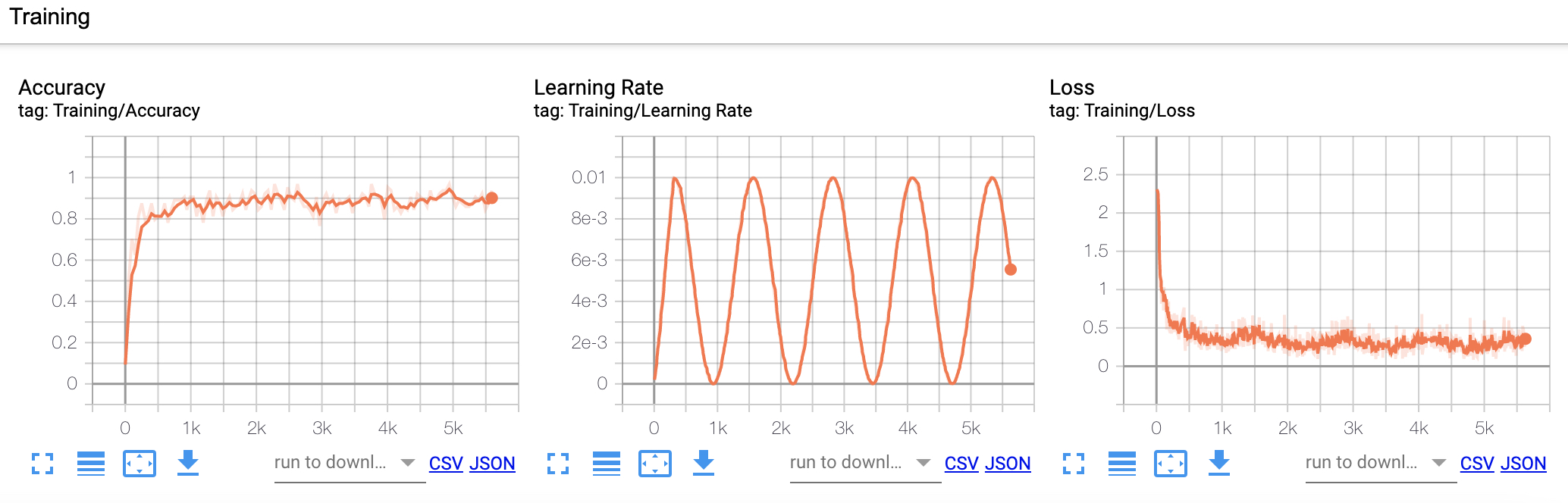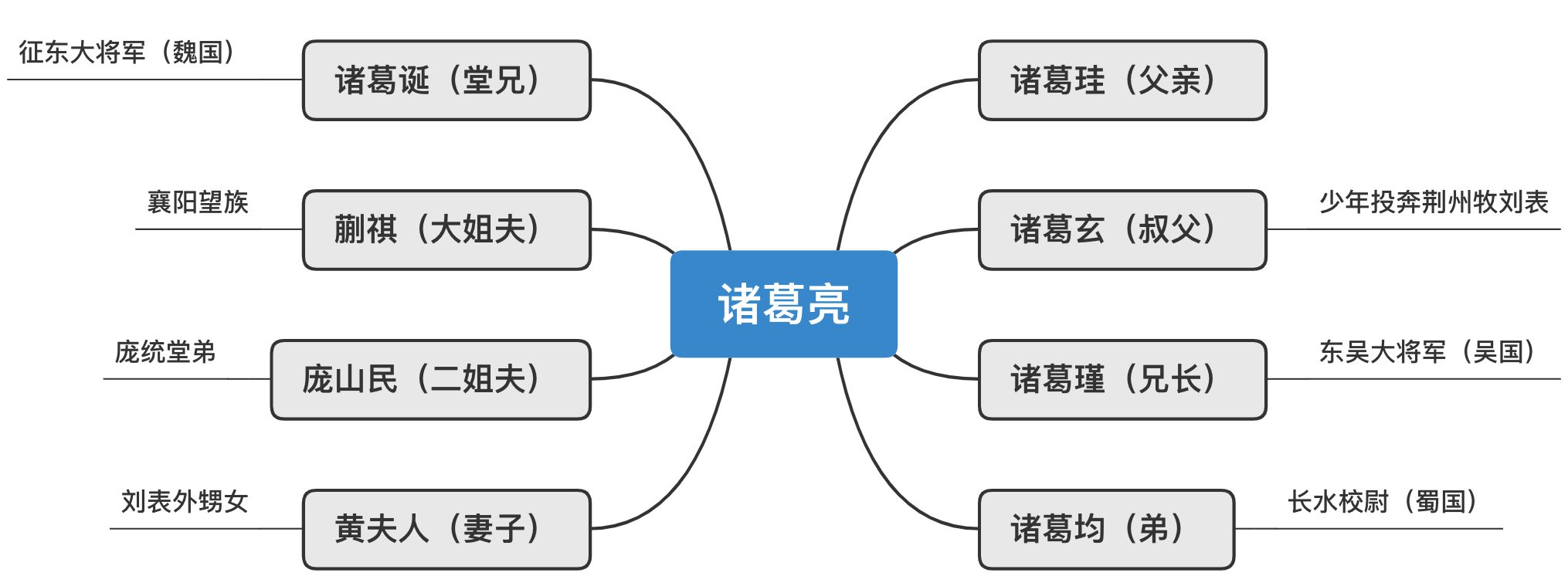### 推荐阅读

 Transformers之自定义学习率动态调整

 LeNet5网络模型详解